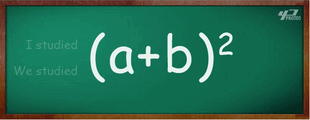Problems
251 - Equation

Equation

Time Limit: 1 sec

The Problem

We all are familiar with the equation (x + y) 2 = X2 + Y2 + 2XY.

The value of m is given. You have to find out the result of (m + (1/m)) 4

Example:

m = 6

Result = 1446.112The Input

Input file will contain a single integer value m (m<=25).

The Output

Output file will print the result rounded to 3 decimal points in a separate line.

Sample Input

6

Sample Output

1446.112

Problem Setter: Samia Safa Ahmed